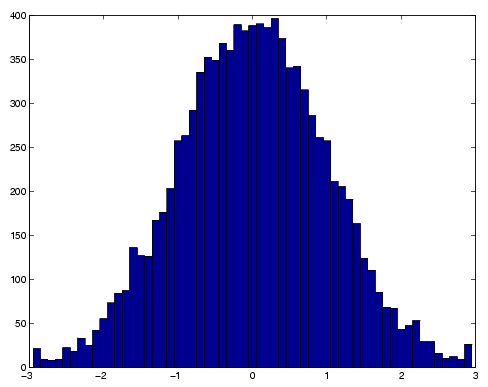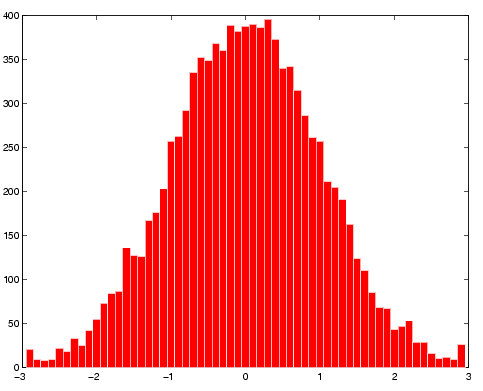MATLAB Function Referencehist

Histogram plot

Syntax

• ```n = hist(Y)
n = hist(Y,x)
n = hist(Y,nbins)
[n,xout] = hist(...)
hist(...)
hist(axes_handle,...)
```

Description

A histogram shows the distribution of data values.

```n = hist(Y) ``` bins the elements in vector `Y` into 10 equally spaced containers and returns the number of elements in each container as a row vector. If `Y` is an `m`-by-`p` matrix, `hist` treats the columns of `Y` as vectors and returns a 10-by-`p` matrix `n`. Each column of `n` contains the results for the corresponding column of `Y`.

```n = hist(Y,x) ``` where `x` is a vector, returns the distribution of `Y` among `length``(x)` bins with centers specified by `x`. For example, if `x` is a 5-element vector, `hist` distributes the elements of `Y` into five bins centered on the x-axis at the elements in `x`. Note: use `histc` if it is more natural to specify bin edges instead of centers.

```n = hist(Y,nbins) ``` where `nbins` is a scalar, uses `nbins` number of bins.

```[n,xout] = hist(...) ``` returns vectors `n` and `xout` containing the frequency counts and the bin locations. You can use `bar``(xout,n)` to plot the histogram.

```hist(...) ``` without output arguments produces a histogram plot of the output described above. `hist` distributes the bins along the x-axis between the minimum and maximum values of `Y`.

```hist(axes_handle,...) ``` plots into the axes with handle `axes_handle` instead of the current axes (`gca`).

Remarks

All elements in vector `Y` or in one column of matrix `Y` are grouped according to their numeric range. Each group is shown as one bin.

The histogram's x-axis reflects the range of values in `Y`. The histogram's y-axis shows the number of elements that fall within the groups; therefore, the y-axis ranges from `0` to the greatest number of elements deposited in any bin.

The histogram is created with a patch graphics object. If you want to change the color of the graph, you can set patch properties. See the "Example" section for more information. By default, the graph color is controlled by the current colormap, which maps the bin color to the first color in the colormap.

Examples

Generate a bell-curve histogram from Gaussian data.

• ```x = -2.9:0.1:2.9;
y = randn(10000,1);
hist(y,x)```

Change the color of the graph so that the bins are red and the edges of the bins are white.

• ```h = findobj(gca,'Type','patch');
set(h,'FaceColor','r','EdgeColor','w')```

See Also

`bar`, `ColorSpec`, `histc`, `patch`, `rose`, `stairs`

Specialized Plotting for related functions

Histograms for examples

© 1994-2005 The MathWorks, Inc.